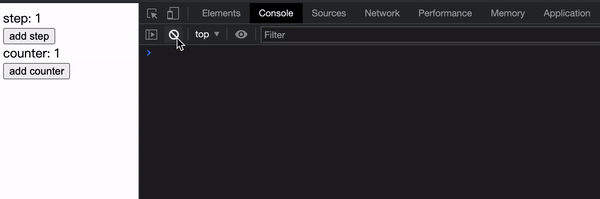# Model Communication#

Model communication refers to communication between different Models, as well as communication between Effects and Actions within the same Model.

## Communication between Models#

Models are not isolated from each other and can communicate with each other. There are mainly two scenarios:

1. Accessing the State and Actions of other Models in the Model.
2. Listening to changes in other Models in the Model.

Here, we will transform the simple counter application in the Quick Start section into a counter application that allows you to set the step frequency. By setting the step frequency, you can affect the magnitude of each counter increase.

We abstract two Models, `stepModel` (step frequency) and `counterModel` (counter):

``````import { model } from '@modern-js/runtime/model';

const stepModel = model('step').define({
state: 1,
});

const counterModel = model('count').define((context, { use, onMount }) => {
const [, , subscribeStep] = use(stepModel);

onMount(() => {
return subscribeStep(() => {
console.log(
`Subscribe in counterModel: stepModel change to \${use(stepModel)}`,
);
});
});

return {
state: {
value: 1,
},
actions: {
const step = use(stepModel);
state.value += step;
},
},
};
});

export { stepModel, counterModel };``````

`stepModel` declares only one `state`, with an initial value of 1.

`counterModel` loads `stepModel` with the `use` function, and retrieves the returned `subscribeStep` function to listen for changes to the `stepModel` state. `onMount` is a hook function that is executed after the Model is mounted. `counterModel` begins to subscribe to the state changes of `stepModel` after it has been mounted, and prints out the latest value of `stepModel`.

`counterModel` accesses `stepModel` using the `use` function, and in the `add` function, the current value of `stepModel` (step frequency) can be obtained to perform increments using this value.

Note

When you need to access the state of another Model, you must call `use` during the actual execution phase of the current Actions or Effects function (in this example, the `add` function) to ensure that the obtained State is the latest value. Therefore, although we also call `use(stepModel)` in the callback function of `define`, we do not destructure the `state` value of `stepModel` because the callback function of `define` is executed during the mounting phase of the Model, and at this time, the `state` of `stepModel` obtained may be different from the value obtained when `add` is executed.

Modify App.tsx:

``````import { useModel } from '@modern-js/runtime/model';
import { counterModel, stepModel } from './models/count';

function Counter() {
const [state, actions] = useModel(counterModel);
const [step, stepActions] = useModel(stepModel);

return (
<div>
<div>step: {step}</div>
<button onClick={() => stepActions.setState(step + 1)}>add step</button>
<div>counter: {state.value}</div>
</div>
);
}

export default function App() {
return <Counter />;
}``````

Modern.js has enabled auto-generate actions by default, so even though there are no Actions defined manually in the `stepModel`, the auto-generated `setState` can still be used.

• Click add counter to trigger the counter to increase.

The final effect is as follows:• Full example code for this section can be found here.

In the previous example of `counterModel`, we called `use` within the Actions function to get other Model objects. If we only need to call Actions of other Models, we can also use `use` to get the Actions of Models in the `define` callback function because Actions are functions and there is no issue of value expiration. For example:

``````const barModel = model('bar').define({
// ..
});

const fooModel = model('foo').define((context, utils) => {
// get barModel actions
const [, actions] = utils.use(barModel);
return {
// ...
effects: {
// ...
// invoke barModel action
barModel.actionA();
},
// ...
// invoke barModel action
barModel.actionB();
},
},
};
});``````

Here, we no longer need to repeatedly get the `barModel` object in `loadA` and `loadB`, which simplifies the code logic.

## Communication within a Model#

Communication within a Model can also be divided into two main scenarios:

1. Effects functions call the Actions functions of the same Model or other Effects functions.
2. Actions functions call other Actions functions of the same Model.

In the Managing Side Effects section, we demonstrated how Effects functions call Actions functions.

Here we provide another example:

``````const fooModel = model('foo').define((context, { use, onMount }) => ({
state: {
a: '',
b: '',
},
actions: {
},
},
},
effects: {
// get current Model actions
const [, actions] = use(fooModel);
const res = await mockFetchA();
actions.setA(res);
},
// get current Model actions
const [, actions] = use(fooModel);
const res = await mockFetchB();
actions.setB(res);
},
},
}));``````

In this example, the two Effects functions of `fooModel` need to call their own Actions functions. Here, we have called `use` once in each Effects function. Why can't we use `use` to get the Actions of the Model itself in the `define` callback function, as in the example of Model communication? This is because when calling `use` to get a Model, it first checks whether the Model has been mounted. If it has not been mounted, the mounting logic will be executed first.

The `define` callback function is executed during the mounting phase, so calling `use` to get the Model itself during the mounting phase will result in an infinite loop (which will throw an error in the actual execution process). Therefore, you must not call `use` in the `define` callback function to get the Model itself.

However, we can use the `onMount` hook function to get the Model itself through `use` after the Model has been mounted:

``````const fooModel = model('foo').define((context, { use, onMount }) => {
let actions;

onMount(() => {
// after fooModel mounted, get actions
[, actions] = use(fooModel);
});

return {
state: {
a: '',
b: '',
},
actions: {
},
},
},
effects: {
const res = await mockFetchA();
actions.setA(res);
},